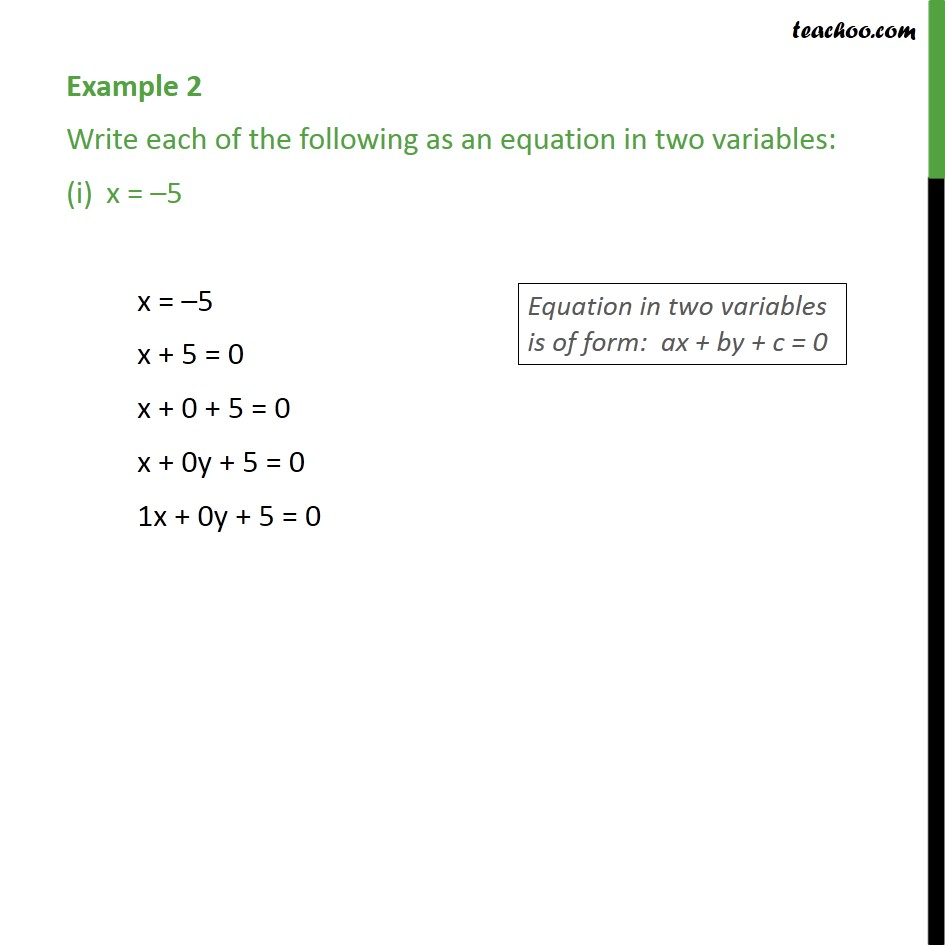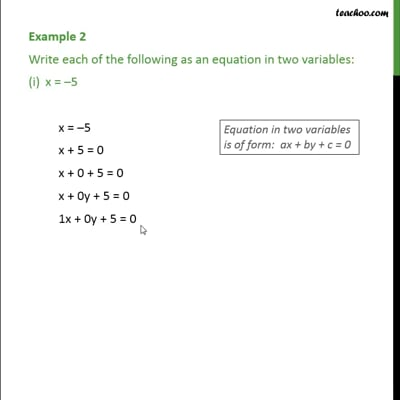Definition and General Form of Linear EquationThis video is only available for Teachoo black users

Introducing your new favourite teacher - Teachoo Black, at only ₹83 per month

### Transcript

Example 2 Write each of the following as an equation in two variables: x = 5 x = 5 x + 5 = 0 x + 0 + 5 = 0 x + 0y + 5 = 0 1x + 0y + 5 = 0